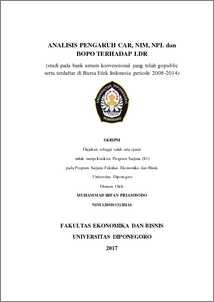# ANALISIS PENGARUH CAR, NIM, NPL dan BOPO TERHADAP LDR (studi pada bank umum konvensional yang telah gopublic serta terdaftar di Bursa Efek Indonesia periode 2008-2014)

PRIAMBODO, Muhammad Irfan and PRASETIONO, Prasetiono (2017) ANALISIS PENGARUH CAR, NIM, NPL dan BOPO TERHADAP LDR (studi pada bank umum konvensional yang telah gopublic serta terdaftar di Bursa Efek Indonesia periode 2008-2014). Undergraduate thesis, Fakultas Ekonomika dan Bisnis.Preview
PDF - Published Version
1192Kb

## Abstract

This research was conducted to examine the influence of the Capital Adequacy Ratio (CAR), Net Interest Margin (NIM), Non Performing Loan (NPL), and Operational Efficiency Ratio toward Loan to Deposit Ratio (LDR). The sample of this research is the conventional commercial bank listed on the Indonesia Stock Exchange period 2008 through 2014. Purposive sampling method were used as samples determining method and 21 banks selected as the sample of this research. The analytical methods used are classical assumptions test consist of normality test, multicolinierity test, autocorellation test, and heteroscedasticity test and multiple regression analysis and hypothesis testing consist of t-statistic and F-statistic at level significance 5%. The results of the research simultantly using F test, showed that size, Capital Adequacy Ratio (CAR), Net Interest Margin (NIM), Non Performing Loan (NPL), and Operational Efficiency Ratio variables influence significantly toward Loan to Deposit Ratio (LDR). Partially varibles using t test, CAR is significant negative influence on the LDR with a significance level owith t value of −0,994 0,000 <0,050. NIM is significant positive influence on the LDR with a significance level of 0,000 < 0,050 and t value of 2,452. NPL is not significance negative influence on the LDR with a significance level of 0,543 > 0,050 and t value is −0,620 and the last variable Operational Efficiency Ratio is significant positive influence on the LDR with a significance level of 0,023 < 0,050 and the t value is 0,205. The coefficient determinant (r square) is 0,254 which means 25,4% LDR variation explained by ,CAR, NPL, NIM and Operational Efficiency Ratio, whereas the other is explained by another variables which was not to be entered in the research model.

Item Type: Thesis (Undergraduate) Capital Adequacy Ratio (CAR), Net Interest Margin (NIM), Non Performing Loan (NPL), Operational Efficiency Ratio , Loan to Deposit Ratio (LDR) Capital Adequacy Ratio (CAR), Net Interest Margin (NIM), Non Performing Loan (NPL), Operational Efficiency Ratio , Loan to Deposit Ratio (LDR) H Social Sciences > H Social Sciences (General) Faculty of Economics and Business > Department of Management 54849 Mr. Perpustakaan Fakultas Ekonomi 17 Jul 2017 10:22 17 Jul 2017 10:22

Repository Staff Only: item control page